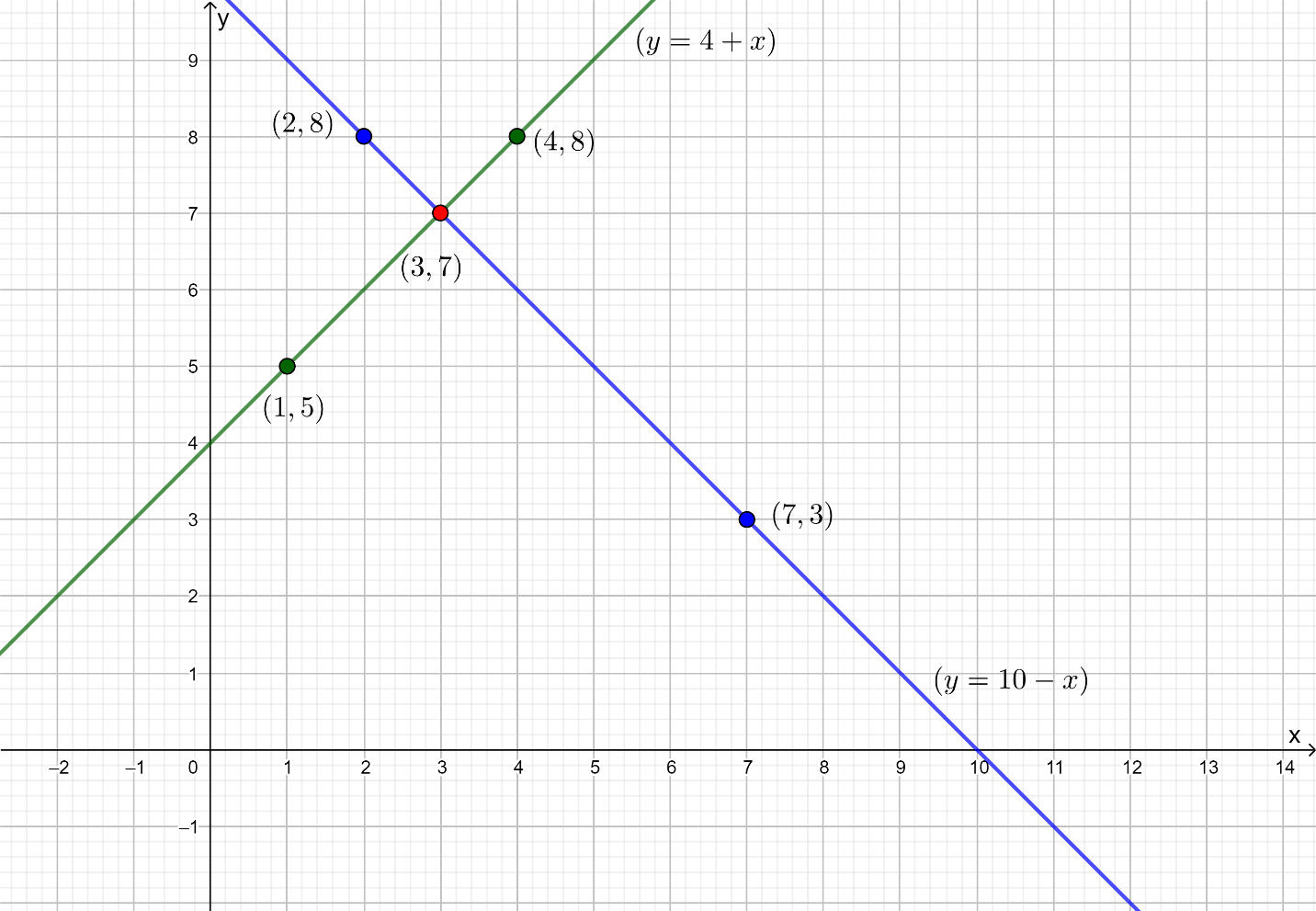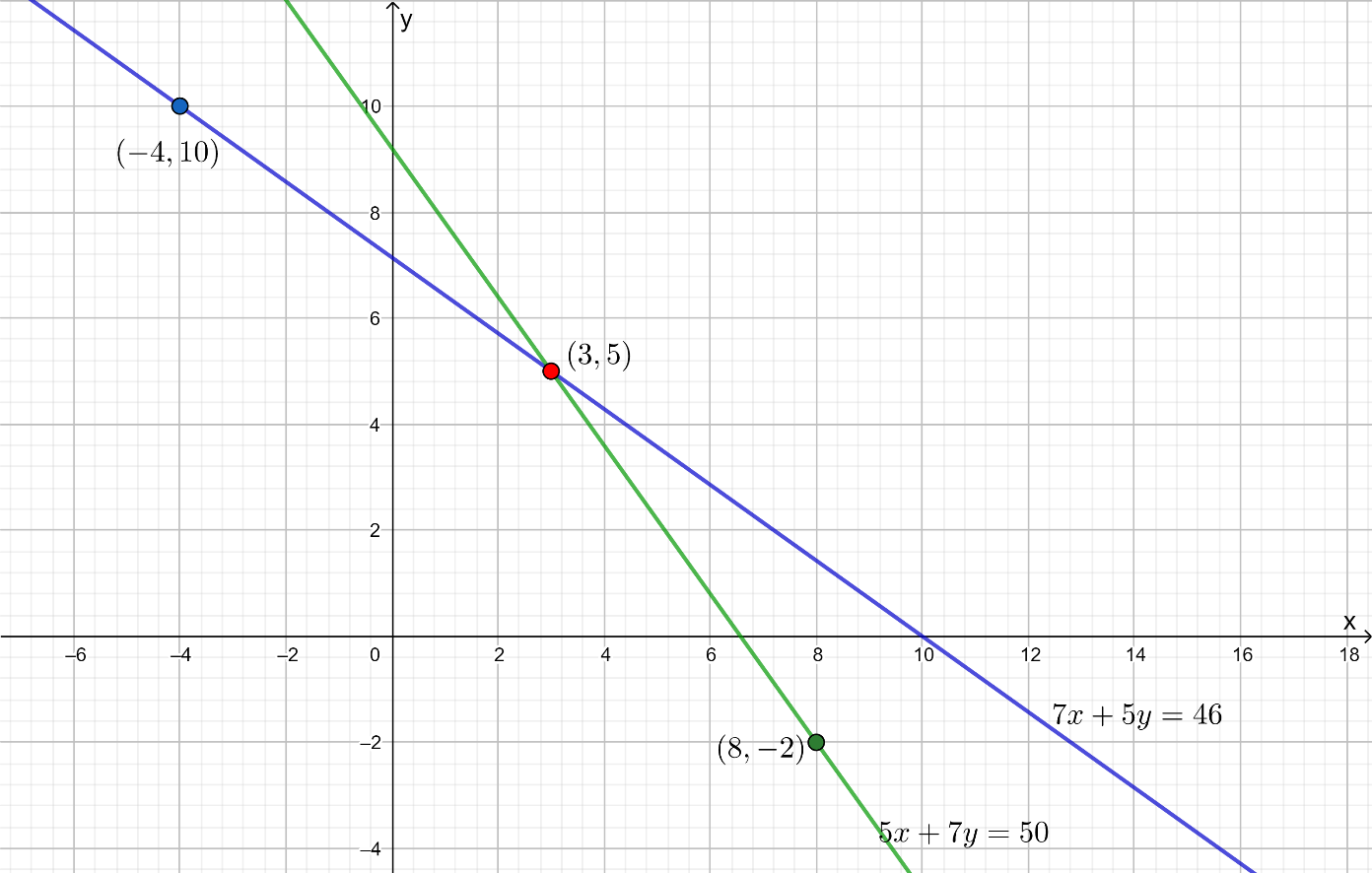# Ex.3.2 Q1 Pair of Linear Equations in Two Variables Solution - NCERT Maths Class 10

Go back to  'Ex.3.2'

## Question

Form the pair of linear equations in the following problems and find their Solutions graphically.

(i) $$10$$ students of Class X took part in a Mathematics quiz. If the number of girls is $$4$$ more than the number of boys, find the number of boys and girls who took part in the quiz.

(ii) $$5$$ pencils and $$7$$ pens together cost ₹ $$50,$$ whereas $$7$$ pencils and $$5$$ pens together cost ₹ $$46.$$ Find the cost of one pencil and that of one pen.

Video Solution
Pair Of Linear Equations In Two Variables
Ex 3.2 | Question 1

## Text Solution

(i)

What is Known?

(i) Number of students took part in Quiz $$= 10$$

(ii) Number of girls is $$4$$ more than number of boys

What is Unknown?

Finding Solutions graphically for the given situation.

Reasoning:

Assuming the number of boys as $$x$$ and the number of girls as $$y,$$ two linear equations can be formed for the above situation.

Steps:

Total number of boys and girls is:

$x + y = 10$

Number of girls is $$4$$ more than the number of boys,

Mathematically:

\begin{align}y &= x + 4\\ - x + y &= 4\end{align}

Algebraic representation where $$x$$ and $$y$$ are the number of boys and girls respectively.

\begin{align}x + y &= 10 \,\dots(1)\\- x + y &= 4\,\dots(2) \end{align}

Therefore, the algebraic representation for equation 1 is:

\begin{align}x + y &= 10\\y &= 10-x\end{align}

And, the algebraic representation is for equation 2 is:

\begin{align} - x + y& = 4\\y &= x + 4\end{align}

Let us represent these equations graphically. For this, we need at least two solutions for each equation. We give these solutions in table shown below.

 $$x$$ $$2$$ $$7$$ $$y = 10 - x$$ $$8$$ $$3$$

 $$x$$ $$1$$ $$4$$ $$y = x + 4$$ $$5$$ $$8$$

The graphical representation is as follows.From graph solution $$\left( {x,y} \right) = \left( {3,7} \right)$$

Number of boys $$= 3$$

Number of girls$$= 7$$

(ii)

What is Known?

(i) $$5$$ pencils and $$7$$ pens cost ₹ $$50$$ $$\left( {x,y} \right) = \left( {3,7} \right)$$

(ii) $$7$$ pencils and $$5$$ pens cost ₹ $$46$$

What is Unknown?

Finding Solutions graphically for the given situation.

Reasoning:

Assuming the cost of $$1$$ pencil as ₹ $$x$$ and the cost of $$1$$ pen as ₹ $$y,$$ two linear equations are to be formed for the above situation.

Steps:

Let us assume cost of $$1$$ pencil be $$x$$ and cost of $$1$$ pen be $$y.$$

The cost of $$5$$ pencils and $$7$$ pens is ₹ $$50.$$

Mathematically,

$5x + 7y = 50$

And, the cost of $$7$$ pencils and 5 pens is ₹ $$50.$$

Mathematically,

$7x + 5y = 46$

Algebraic representation where $$x$$ and $$y$$ are the cost of $$1$$ pencil and $$1$$ pen respectively.

\begin{align}5x + 7y &= 50 \,\dots (1) \\7x + 5y &= 46 \,\dots(2) \end{align}

Therefore, the algebraic representation for equation $$1$$ is:

\begin{align}5x+ 7y &= 50\\7y &= 50-5x\\y &= \frac{{50 - 5x}}{7}\end{align}

And, the algebraic representation for equation $$2$$ is:

\begin{align}7x + 5y &= 46\\5y &= 46-7x\\y &= \frac{{46 - 7x}}{5}\end{align}

Let us represent these equations graphically. For this, we need at least two solutions for each equation. We give these solutions in table shown below.

 $$x$$ $$3$$ $$- 4$$ $$y = \frac{{50 - 5x}}{7}$$ $$5$$ $$10$$

 $$x$$ $$3$$ $$8$$ $$y = \frac{{46 - 7x}}{5}$$ $$5$$ $$- 2$$From graph Solution $$\left( {x,y} \right) = \left( {3,5} \right)$$

Cost of one pencil $$=$$$$3$$

Cost of one pen $$=$$$$5$$

Learn from the best math teachers and top your exams

• Live one on one classroom and doubt clearing
• Practice worksheets in and after class for conceptual clarity
• Personalized curriculum to keep up with school